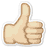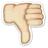Meaning Benefit-cost ratio
What does Benefit-cost ratio mean? Here you find 9 meanings of the word Benefit-cost ratio. You can also add a definition of Benefit-cost ratio yourself

# 1

00# Benefit-cost ratio

An analytical tool used in public planning; a ratio of total measurable benefits divided by the initial capital cost. Also see: Cost Benefit Analysis.
 Source: inboundlogistics.com

# 2

00# Benefit-cost ratio

The benefit of an activity per dollar of cost. Benefit-cost ratios (or alternatively cost-benefit ratios) are frequently estimated for many forms of government spending, as well as a growing number of [..]
 Source: glossary.econguru.com

# 3

00# Benefit-cost ratio

An analytical tool used in public planning; a ratio of total measurable benefits divided by the initial capital cost. see Cost Benefit Analysis.
 Source: logisuite.com

# 4

00# Benefit-cost ratio

see Profitability index
 Source: financeisland.com

# 5

00# Benefit-cost ratio

The value of benefits divided by the cost of a project or alternative. By definition includes only those benefits that can be expressed in financial terms
 Source: ita-aites.org

# 6

00# Benefit-cost ratio

The ratio of a project's total benefits to total costs, measured in monetary net present value. In benefit-cost analysis, alternative projects are incrementally compared to find the greatest net [..]
 Source: its.uci.edu

# 7

00# Benefit-cost ratio

The economic value of the reduction in fatalities, injuries, and property damage divided by the cost of the collision-reducing measure.
 Source: ite.org

# 8

00# Benefit-cost ratio

An analytical tool used in public planning; a ratio of total measurable benefits divided by the initial capital cost.  see Cost Benefit Analysis.
 Source: vpa.org.vn

# 9

00# Benefit-cost ratio

This summary measure directly compares benefits and costs. To calculate, divide total discounted benefits by total discounted costs. A BCR greater than 1 means the benefits outweigh the costs and the investment should be considered. If the ratio is less than 1, the costs outweigh the benefits. If the BCR is equal to 1, the benefits equal the costs. [..]
 Source: cbkb.org

 Add meaning of Benefit-cost ratio Word count:
 Name: E-mail: (* optional)

 << Appropriation Branch line >>

Dictionary.university is a dictionary written by people like you and me.